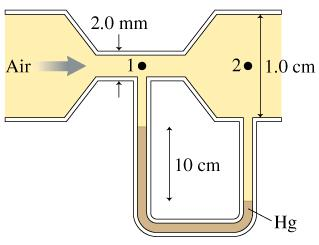# Problem: Air flows through the tube shown in the figure. Assume that air is an ideal fluid. ﻿v1 = 149 m/sWhat is the air speed v2 at point 2?

###### FREE Expert Solution

Continuity equation:

$\overline{){\mathbf{Q}}{\mathbf{=}}{{\mathbf{A}}}_{{\mathbf{1}}}{{\mathbf{v}}}_{{\mathbf{1}}}{\mathbf{=}}{{\mathbf{A}}}_{{\mathbf{2}}}{{\mathbf{v}}}_{{\mathbf{2}}}{\mathbf{=}}{{\mathbf{A}}}_{{\mathbf{n}}}{{\mathbf{v}}}_{{\mathbf{n}}}}$

Using continuity:

v1A1 = v2A2

v1π(d1/2)2 = v2π(d2/2)2

84% (147 ratings)###### Problem Details

Air flows through the tube shown in the figure. Assume that air is an ideal fluid. ﻿v1 = 149 m/sWhat is the air speed v2 at point 2?